Tags: example nirs

# Analyzing NIRS data recorded during listening to and repeating speech

This example script demonstrates the analysis of data that is shared by Jessica Defenderfer and Aaron Buss in fNIRS data files for event-related vocoding/background noise study.

In the shared data the authors refer to an article “Examining the hemodynamic response during perception of noise-vocoded speech and speech in background noise: An image-based fNIRS study”. However, I have not been able to find an article with precisely that title, althoug another article from the same authors has a title that comes close. The following links point to the shared data and to the published PDF manuscript that explains (some aspects of) the shared data.

To explain the dataset, I will repeat/summarise some of the key features of the PDF manuscript.

The authors write that they examined cortical activity and speech perception during three listening conditions: speech in quiet (SIQ), speech in noise (SIN) & eight-channel vocoded speech (VOC). Thirty-one normal-hearing listeners participated in this study.

The shared data includes files that are named from S1001 to S1040, suggesting that there were 40 participants. There are no data files for subject 2 and 15, hence the shared data set comprises 38 participants which is still more than the 31 mentioned in the PDF paper.

The NIRS headband was developed to secure two light sources and four light detectors over each hemisphere resulting in six measurable channels (30 mm in length) over each hemisphere. So we expect 6 channels, times two hemispheres is 12 channels, times two wavelengths (or HbO and HbR) is 24 channels.

However, as we will see below, the shared dataset contains 28 NIRS channels, which is not consistent with the description in the PDF manuscript.

The paper writes that the experiment uses an event-related design, however the stimuli anr not randomized but are presented in blocks. Condition blocks were randomized (over subjects) to rule out any effect of order, and all sentences of one condition were presented together. Each listening condition (block) began with three practice sentences for familiarization followed by 20 randomized sentences, for a total of 69 trials across all three conditions.

Below we will see that the shared data does not match 3 contitions/blocks with 69 trials each.

Sentences were digitally isolated from their respective lists into 3-second trials using Audacity. The 3-second trials allowed for a variable silence before and after the sentence presentation.

The trial structure in the experiment (see Figure 1 in the PDF manuscript) was as follows

• 500 ms slicence
• 3000 ms sentence presentation
• 500-2000 ms silence
• short audible click, followed by 3000 ms within which the participant repeats the sentence
• 1000-2000 ms silence

That means that a complete trial takes between 0.5+3+0.5+3+1=8 up to 0.5+3+2+3+2=10.5 seconds, after which the next trial starts.

The structure in the trials and the timing as described in the PDF manuscript is consistent with the shared data, suggesting that a similar - although not exactly the same - experiment was performed.

## Building a MATLAB analysis script

``````filenames = {
'S1001_run01.nirs'
'S1003_run01.nirs'
'S1004_run01.nirs'
'S1005_run01.nirs'
'S1006_run01.nirs'
'S1007_run01.nirs'
'S1008_run01.nirs'
'S1009_run01.nirs'
'S1010_run01.nirs'
'S1011_run01.nirs'
'S1012_run01.nirs'
'S1013_run01.nirs'
'S1014_run01.nirs'
'S1016_run01.nirs'
'S1017_run01.nirs'
'S1018_run01.nirs'
'S1019_run01.nirs'
'S1020_run01.nirs'
'S1021_run01.nirs'
'S1022_run01.nirs'
'S1023_run01.nirs'
'S1024_run01.nirs'
'S1025_run01.nirs'
'S1026_run01.nirs'
'S1027_run01.nirs'
'S1028_run01.nirs'
'S1029_run01.nirs'
'S1030_run01.nirs'
'S1031_run01.nirs'
'S1032_run01.nirs'
'S1033_run01.nirs'
'S1034_run01.nirs'
'S1035_run01.nirs'
'S1036_run01.nirs'
'S1037_run01.nirs'
'S1038_run01.nirs'
'S1039_run01.nirs'
'S1040_run01.nirs'
};

filename = filenames{1};
``````

## Exploring the files that hold the NIRS data

The authors have shared the data in the Homer `.nirs` format. This format is directly supported by FieldTrip and you can use both the low-level and the high-level FieldTrip functions to read and/or process the data. See the getting started with Homer page for details.

We will first use the low-level reading functions to check on some basic characteristics of the data on disk.

``````hdr = ft_read_header(filename);
``````

## Look at the optode positions

FieldTrip uses the `opto` structure (see ft_datatype_sens) to describe the physical characteristics of the sensor array, and the `layout` structure (see ft_prepare_layout and the plotting and layout tutorials) to describe how the channel-level results are to be plotted schematically in 2D on the screen.

``````optoR = opto;
optoR.optopos(strcmp(opto.optotype, 'transmitter'),:) = nan;
optoT = opto;

figure
hold on
ft_plot_sens(optoR, 'opto', true, 'optoshape', 'sphere', 'label', 'label', 'facecolor', 'r') % red = receivers
ft_plot_sens(optoT, 'opto', true, 'optoshape', 'sphere', 'label', 'label', 'facecolor', 'b') % blue = transmitters
ft_plot_sens(opto, 'opto', false, 'optoshape', 'sphere', 'label', 'label', 'facecolor', 'g') % green = channels, these are in between
``````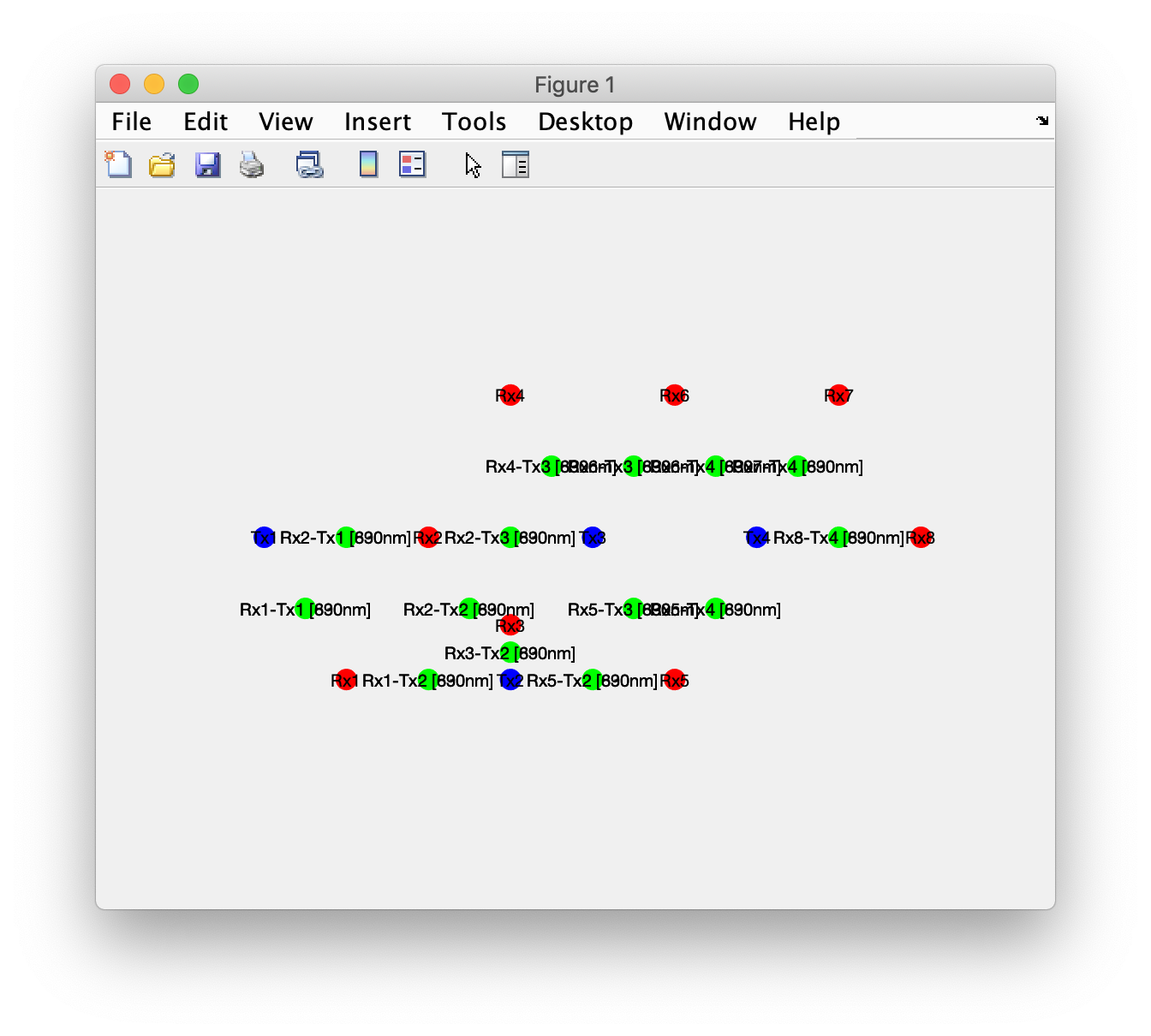Using the rotate option in the MATLAB figure you can look it in 3D.

The spatial arrangement of optodes does not match Figure 2 in the PDF manuscript. Furthermore there are 28 channels (14 at 690nm and 14 at 830nm) rather than 24. The number of transmitters (4) and receivers (8) matches, but the number of channels formed from all possible transmitter-receiver pairs is slightly higher. Since figure 2 in the PDF manuscript only labels the channels in numerical order and does not name or number the optodes, we cannot really tell which channel corresponds to which location on the scalp or over the brain.

## Reading in the continuous NIRS data

``````cfg = [];
cfg.dataset = filename;
data_raw = ft_preprocessing(cfg);
``````

If you look in data_raw.label, you can see that there are 28 NIRS channels, 8 “aux” channels and one “s” channel that represents the stimuli. See the Homer documentation for a description of the file format.

Let us have a look at the NIRS data

``````cfg = [];
cfg.viewmode = 'vertical';
cfg.preproc.demean = 'yes';
cfg.blocksize = 60; % show the data in blocks of 60 seconds
cfg.channel = 'nirs';
ft_databrowser(cfg, data_raw)
``````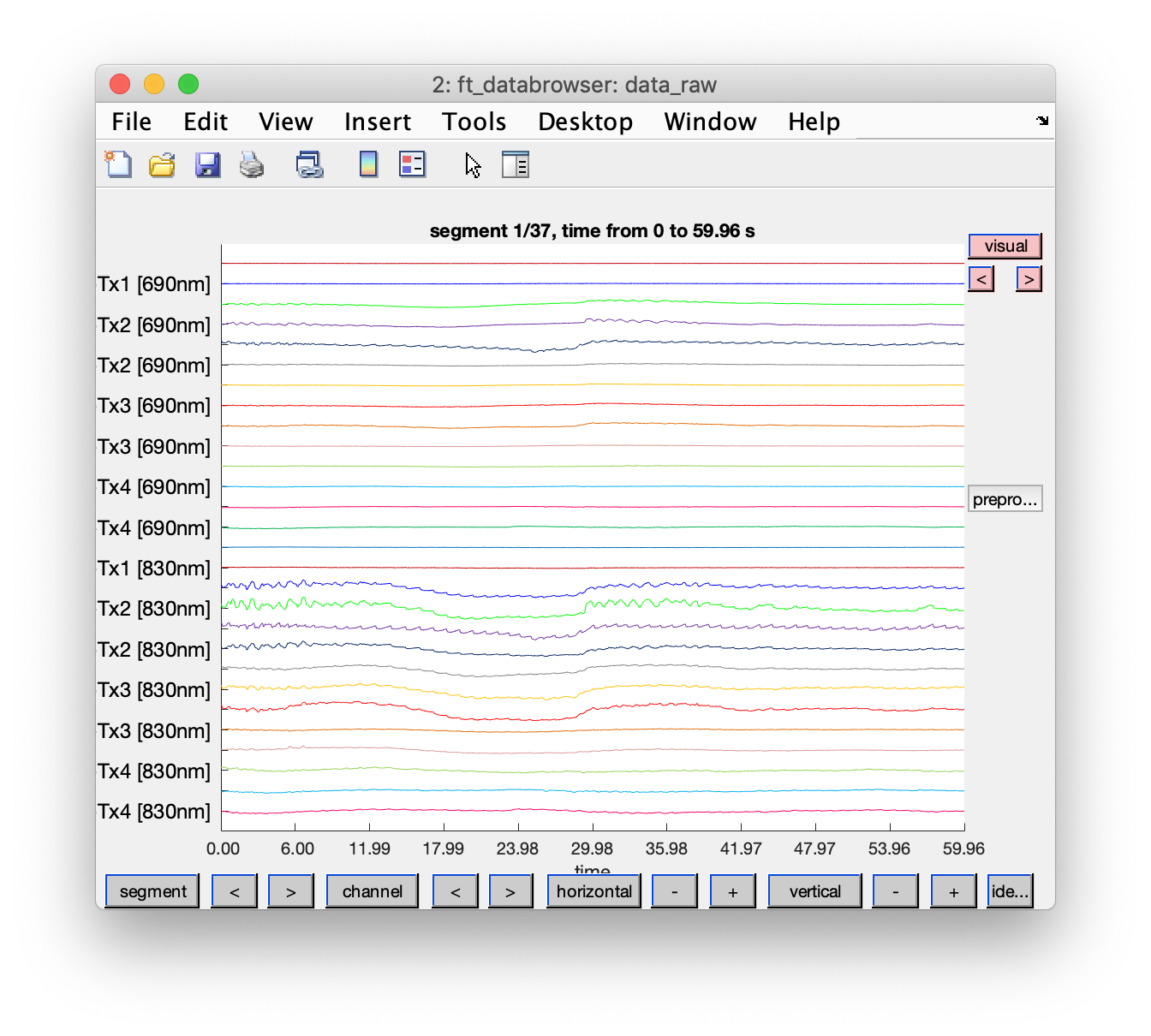## Examining the TTL triggers and events

Let us have a look at the triggers, together with a single NIRS channel for comparison. Using ft_databrowser I already identified that the “s” channel (with the stimulus accordimng to the `.nirs` format) does not contain anything. The channels “aux1” and “aux2” are interesting.

``````cfg = [];
cfg.viewmode = 'vertical';
cfg.preproc.demean = 'yes';
cfg.channel = {'Rx1-Tx1 [690nm]', 'aux1', 'aux2'};
cfg.blocksize = hdr.nSamples/hdr.Fs; % show the whole recording at once
cfg.ylim = [-10000 10000]*3;
cfg.mychan = {'aux1', 'aux2'};   % with mychan/mychanscale we can scale these channels to better match the amplitudes of the NIRS channels
cfg.mychanscale = [10000 10000]; % this was actually determined further down in the script, but reused here
ft_databrowser(cfg, data_raw)
``````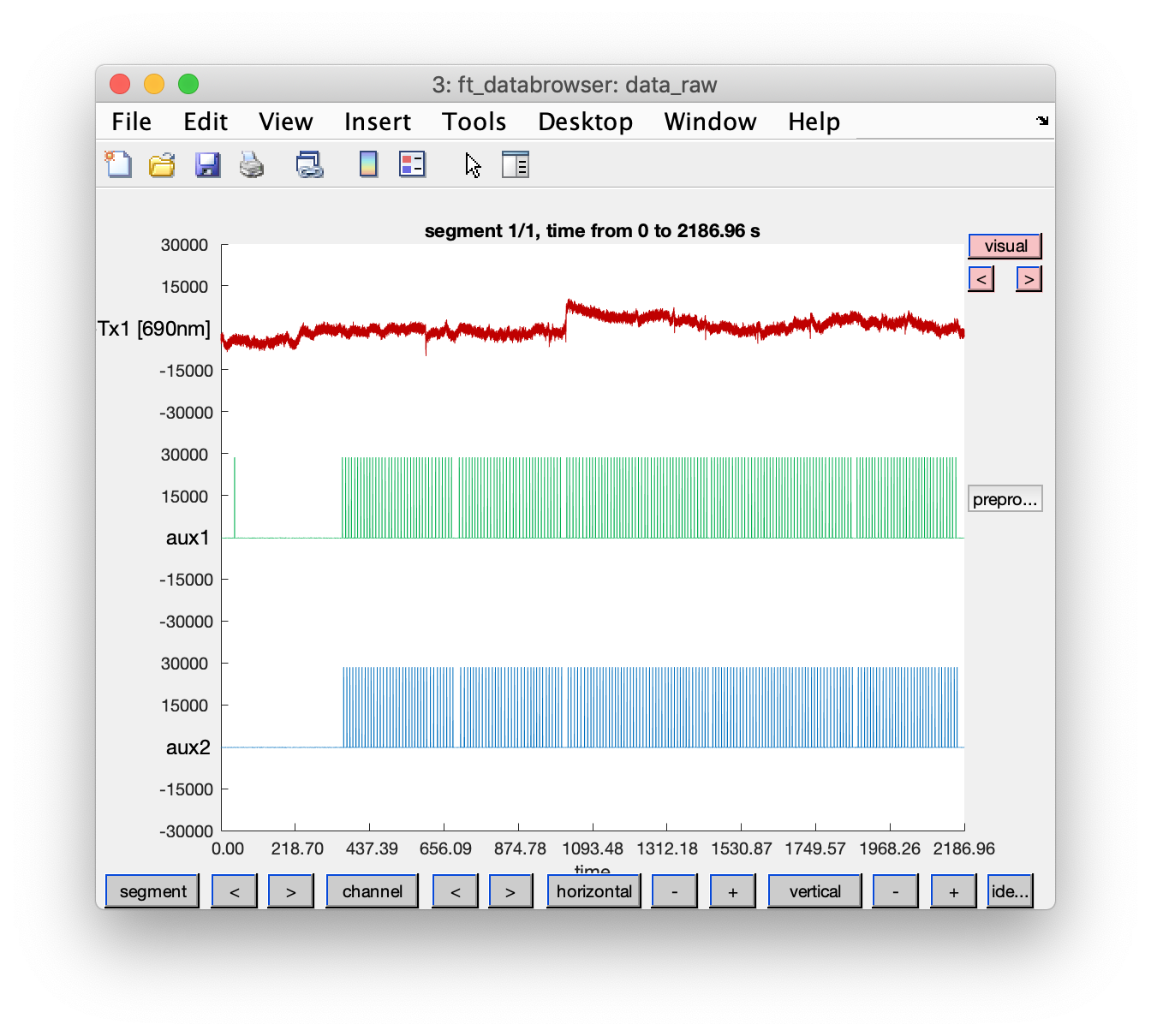Using the “channel” button at the bottom of the screen we can make a different selection of channels to be displayed.

The stimulus channel does not contain any events, the “aux1” and “aux2” channels contain analog TTL pulses. There is an aux1 trigger all the way at the start, well before the regular sequence starts. We can see that “aux1” and “aux2” are alternating, there is about 4 seconds (with some jitter) between successive triggers in these two channels, which matches the desciption of the trial structure in the PDF manuscript. The alternating pattern starts after about 350 seconds, which is about 6 minutes into the recording.

My interpretation of this sequence of TTL pulses in the “aux1” and “aux2” channels is that aux1 codes for the onset of the sentence being played and aux2 codes for the click that cues the participant to repeat the sentence.

## Identifying the experimental conditions/blocks

The “aux1” and “aux2” channel provide information about the onset of the sentences and the clicks (to cue the response), but they do not convey the condition of each sentence that was presented. From the PDF manuscript we know that the different conditions were presented in blocks.

``````figure
hold on
plot(data_raw.time{1}, data_raw.trial{1}(29:30,:))
legend(data_raw.label(29:30))
xlabel('time (s)');
ylabel('aux value (a.u.)');
``````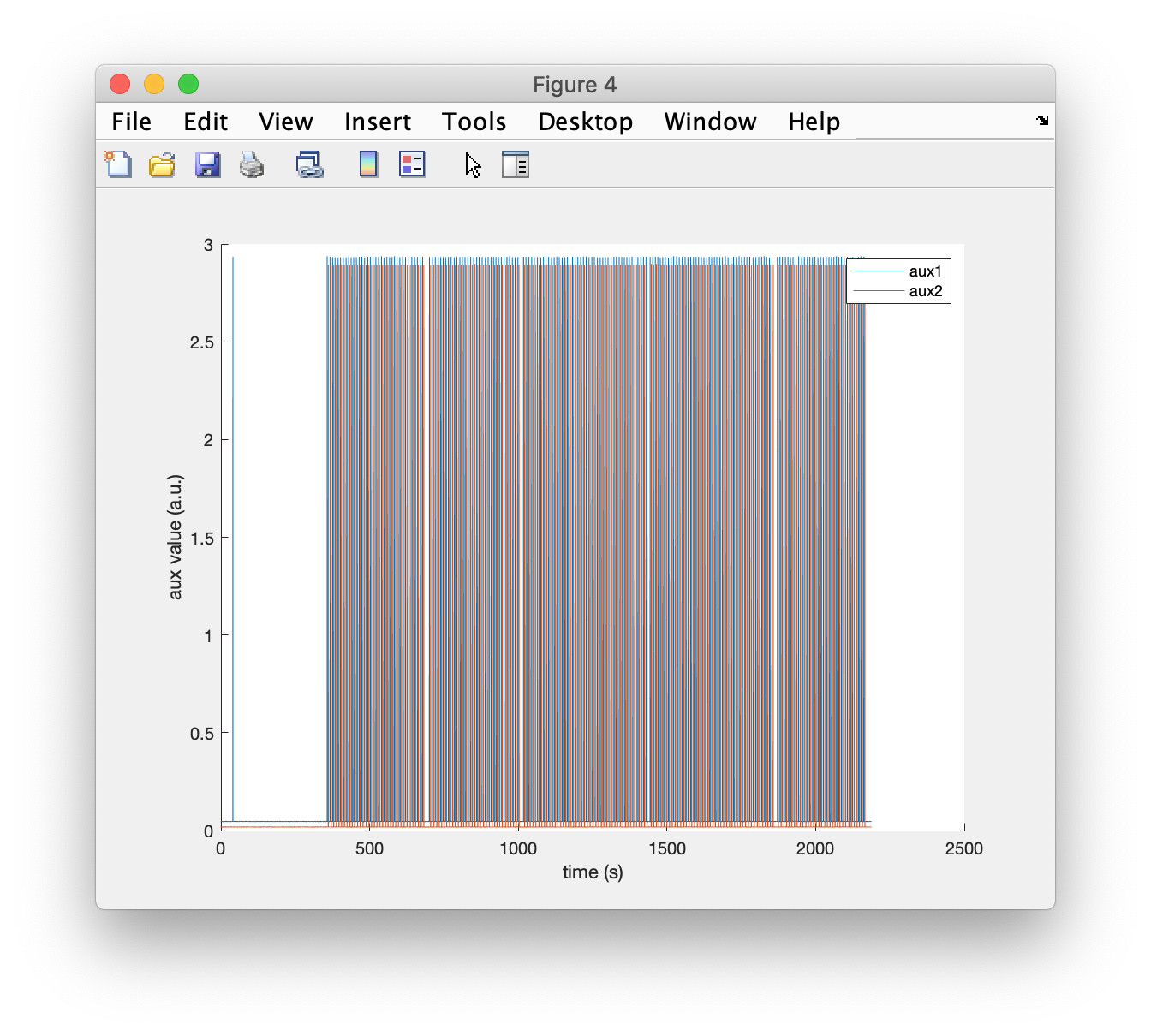There are 5 blocks of events that can be seen, and a single TTL pulse on “aux1” all the way at the start. This is inconsistent with the description of the experimental design in the paper which mentions three conditions; furthermore the number of events per block is inconsistent (69 according to the paper).

By computing the time between successive events, we can find the boundaries between the blocks; the number of samples between subsequent events is larger there.

``````sel = strcmp({event.type}, 'aux1');
aux1 = event(sel);
figure; plot(diff([aux1.sample]));
xlabel('event number')
ylabel('number of samples between events')
``````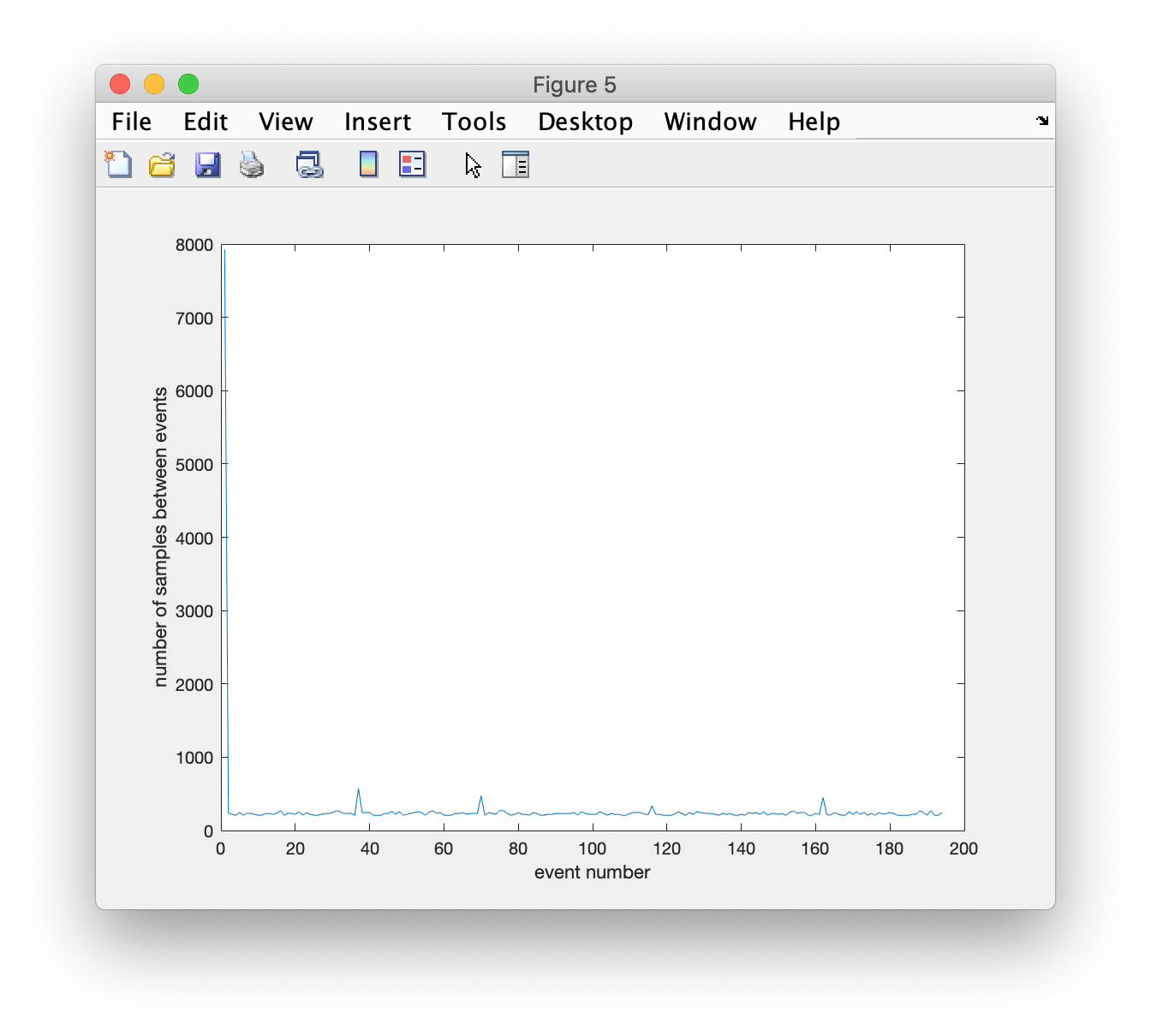There are 5 small peaks to be seen, and a big one immediately following the first event.

``````boundary = find(diff([aux1.sample])>300);       % these are the aux1 events _after_ which a longer pause happens
boundary = [aux1(boundary).sample] + 10*hdr.Fs; % express the boundary in samples, 10 seconds after the event
``````

## Segmenting the continuous data into trials locked to sentence presentation

Let us cut the continuous data into segments corresponding to the trials. The trial structure in the experiment (see Figure 1 in the PDF manuscript) was as follows

• 500 ms slicence
• 3000 ms sentence presentation
• 500-2000 ms silence
• audible click, followed by 3000 ms within which the participant repeats the sentence
• 1000-2000 ms silence

That means that a complete trial takes between 0.5+3+0.5+3+1=8 up to 0.5+3+2+3+2=10.5 seconds, after which the next trial starts.

We will define segments in the data using a `prestim` and `poststim` time that will cause some overlap of subsequent trials.

``````cfg = [];
cfg.trialdef.prestim = 1;     % this goes a bit before the trial starts
cfg.trialdef.poststim = 10.5; % this goes a bit after the trial ends
cfg.dataset = filename;
cfg.trialdef.eventtype = {'aux1'};
cfg1 = ft_definetrial(cfg);

cfg1.trl(1,:) = []; % remove the first one
``````

Since I suspect that the stimulus condition is different in each of the blocks, for each trial I want to code in which block it was.

``````sample = cfg1.trl(:,1) - cfg1.trl(:,3);
block1 = find(sample>boundary(1) & sample<boundary(2));
block2 = find(sample>boundary(2) & sample<boundary(3));
block3 = find(sample>boundary(3) & sample<boundary(4));
block4 = find(sample>boundary(4) & sample<boundary(5));
block5 = find(sample>boundary(5) & sample<inf);
``````

We can look at the number of trials per block (or condition):

``````numel(block1)
ans =
36
numel(block2)
ans =
33
numel(block3)
ans =
46
numel(block4)
ans =
46
numel(block5)
ans =
33
``````

Again this does not match the PDF manuscript, which mentions three blocks of 96 trials each. When we do the same for the 2nd dataset, we recognize the same number of trials in the different blocks, but they occur in another order (46, 36, 46, 33, 33). This is consistent with the description in the PDF manuscript that condition blocks were randomized over subjects. So it seems that the experiment described in the PDF document is very similar to, but not exactly the same, as the one in the shared data. We do not know which 5 conditions were employed in the shared dataset; we also do not know in which order they were presented to each of the subjects. If each of the blocks would have had a unique number of trials (and indentical over subjects), then we could at least have matched the blocks over subjects. Now we have two blocks with 46 and two blocks of 33 trials, which makes matching the (unknown) conditions over subjects impossible. The only condition/block that can be matched over subjects is the one with 36 trials.

Let me plot the TTL channels and the timepoints at which I think the onsets of the sentences is in each condition.

``````figure; hold on
plot(data_raw.time{1}, data_raw.trial{1}(29:30,:))
plot(data_raw.time{1}(sample(block1)), 2.9*ones(size(block1)), 'kx')
plot(data_raw.time{1}(sample(block2)), 2.9*ones(size(block2)), 'mx')
plot(data_raw.time{1}(sample(block3)), 2.9*ones(size(block3)), 'cx')
plot(data_raw.time{1}(sample(block4)), 2.9*ones(size(block4)), 'yx')
plot(data_raw.time{1}(sample(block5)), 2.9*ones(size(block5)), 'gx')
``````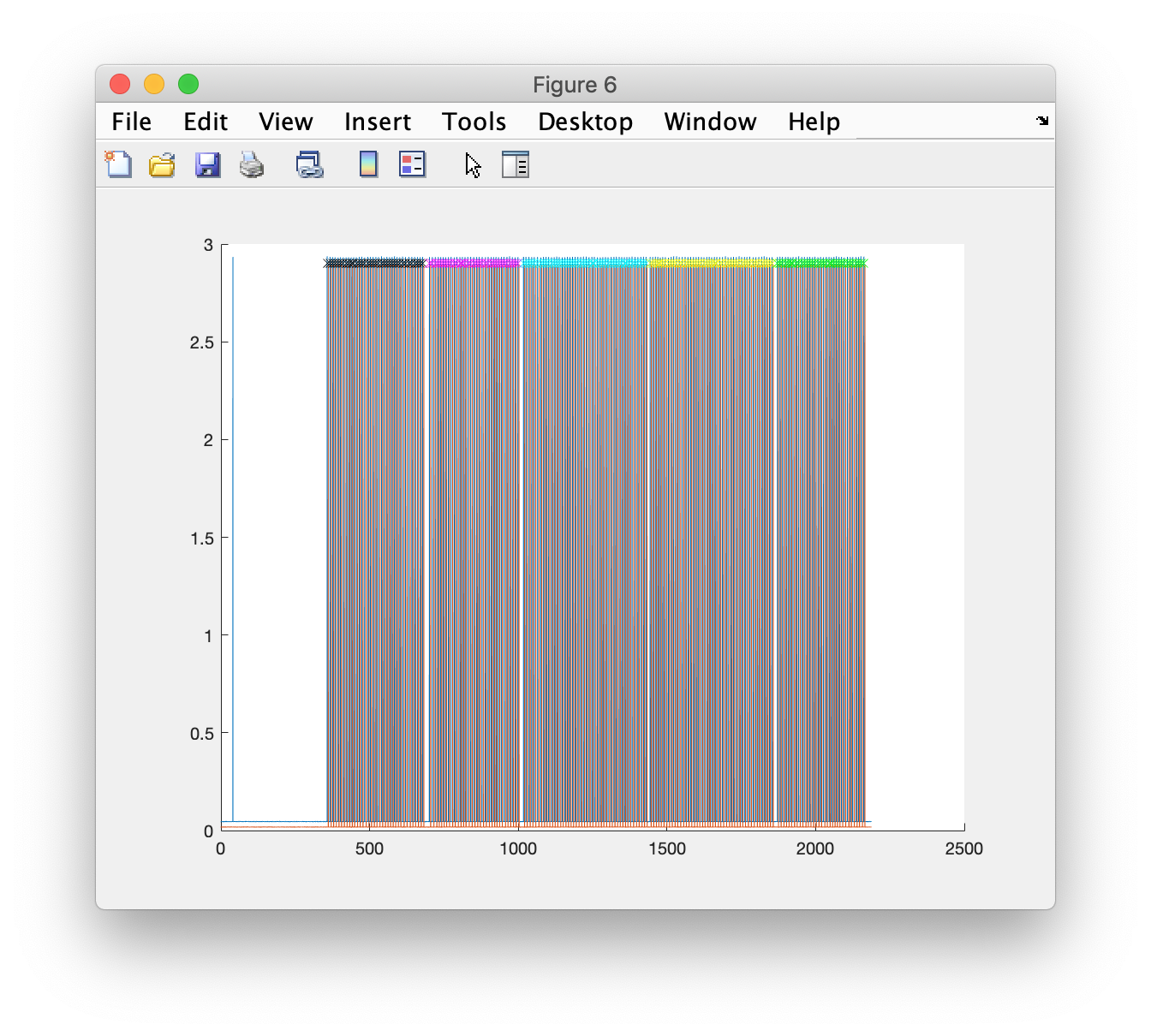By zooming in in the MATLAB figure, we can see that we have correctly identified each TTL pulse onset in each block. The markers in the figure show in different colors that we can associate each trial to one of the blocks or conditions.

The first three sentences are supposed to be trial sentences according to the manuscript, but those cannot be recognized from the trigger sequence (or number). Something to think about is whether the first three events from each block should be excluded from further analysis. For now we will keep them in.

We want to be able to tell the trials in each blocks apart from each other. We will use the codes 11, 12, 23, 14, 15 for the TTL pulse in “aux1” (the stimulus onset) in each of the blocks , and 21, 22, 23, 24, 25 for the TTL pulse in “aux2” (the response onset) in each of the blocks.

``````cfg1.trl(block1,4) = 11; % re-code the values in the 4th column
cfg1.trl(block2,4) = 12; % re-code the values in the 4th column
cfg1.trl(block3,4) = 13; % re-code the values in the 4th column
cfg1.trl(block4,4) = 14; % re-code the values in the 4th column
cfg1.trl(block5,4) = 15; % re-code the values in the 4th column
``````

## Segmenting the continuous data into trials locked to responses

We will do the same for the data segments locked to the response onsets.

``````cfg = [];
cfg.trialdef.prestim = 1;     % this goes a bit before the trial starts
cfg.trialdef.poststim = 10.5; % this goes a bit after the trial ends
cfg.dataset = filename;
cfg.trialdef.eventtype = {'aux2'};
cfg2 = ft_definetrial(cfg);

sample = cfg2.trl(:,1) - cfg2.trl(:,3);
block1 = find(sample>boundary(1) & sample<boundary(2));
block2 = find(sample>boundary(2) & sample<boundary(3));
block3 = find(sample>boundary(3) & sample<boundary(4));
block4 = find(sample>boundary(4) & sample<boundary(5));
block5 = find(sample>boundary(5) & sample<inf);
``````

I think these are the onsets of the clicks in each condition, which trigger the response

``````figure; hold on
plot(data_raw.time{1}, data_raw.trial{1}(29:30,:))
plot(data_raw.time{1}(sample(block1)), 2.8*ones(size(block1)), 'ko')
plot(data_raw.time{1}(sample(block2)), 2.8*ones(size(block2)), 'mo')
plot(data_raw.time{1}(sample(block3)), 2.8*ones(size(block3)), 'co')
plot(data_raw.time{1}(sample(block4)), 2.8*ones(size(block4)), 'yo')
plot(data_raw.time{1}(sample(block5)), 2.8*ones(size(block5)), 'go')
``````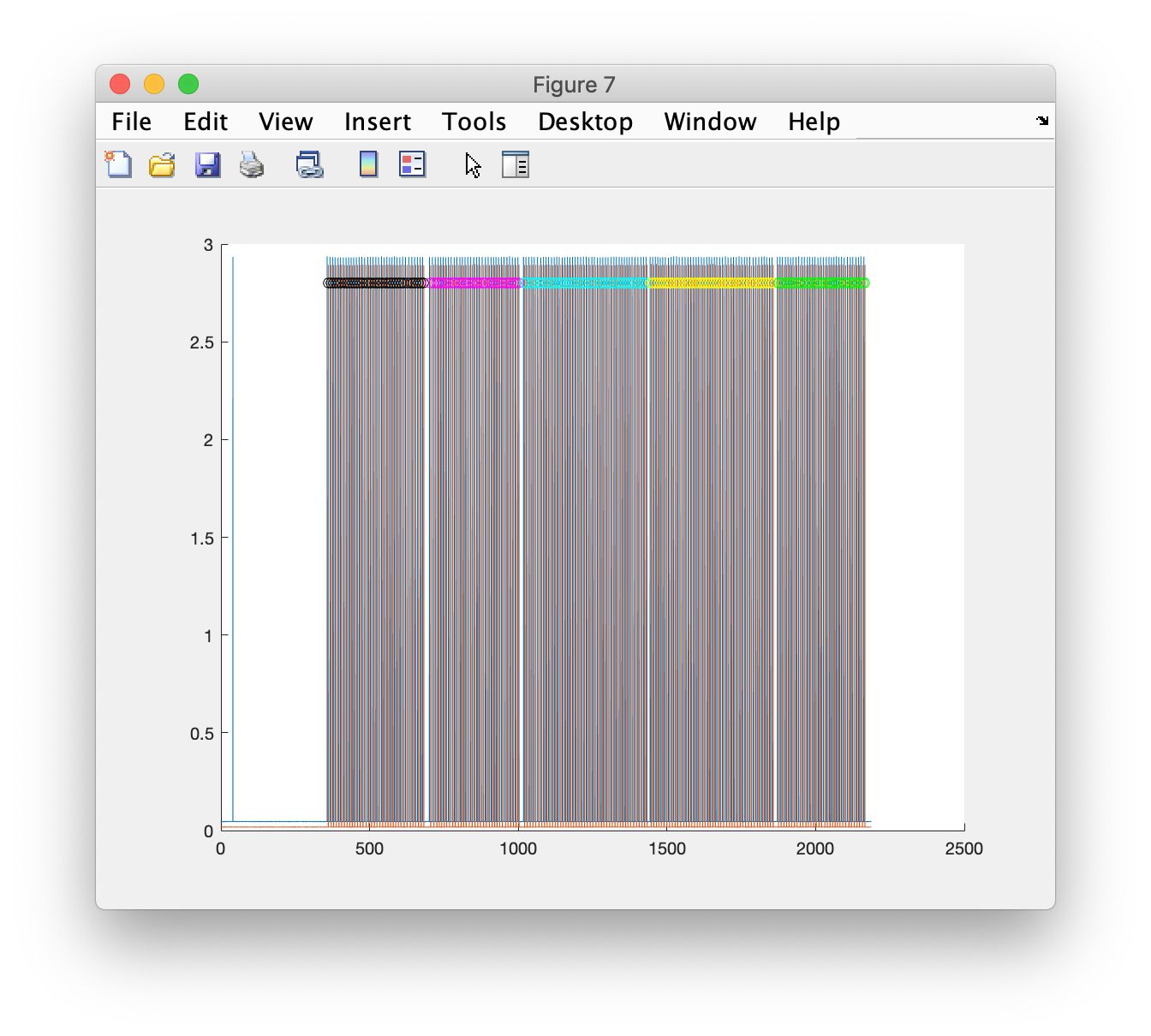``````cfg2.trl(block1,4) = 21; % re-code the values in the 4th column
cfg2.trl(block2,4) = 22; % re-code the values in the 4th column
cfg2.trl(block3,4) = 23; % re-code the values in the 4th column
cfg2.trl(block4,4) = 24; % re-code the values in the 4th column
cfg2.trl(block5,4) = 25; % re-code the values in the 4th column
``````

## Filter and segment the confinuous data

Prior to segmenting the data we can apply a bandpass filter.

``````cfg = [];
cfg.bpfilter = 'yes';
cfg.bpfreq = [0.02 0.8];
cfg.bpfiltord = 2;
cfg.channel = {'nirs'}; % only on the NIRS channels
data_filt = ft_preprocessing(cfg, data_raw);
``````

Do not filter the aux channels, but append them as they are.

``````cfg = \[];
cfg.channel = {'aux1', 'aux2'};
data_aux = ft_selectdata(cfg, data_raw);

cfg = [];
data_filt = ft_appenddata(cfg, data_filt, data_aux);
``````

Now we can segment the data. Since the condition codes for each trial are different, we can simply put them all together. For the following analysis it does not matter that the subsequent trials have quite some overlap, since we will compute conditional averages.

``````cfg = [];
cfg.trl = cat(1, cfg1.trl, cfg2.trl); % the concatenated trials are not in the original order
[dum, order] = sort(cfg.trl(:,1));    % sort them to restore the original order according to the recording
cfg.trl = cfg.trl(order,:);
data_segmented = ft_redefinetrial(cfg, data_filt);
``````

## Convert optical densities into HbO and HbR

Now we convert from optical densities into HbO and HbR concentrations, this uses some functions from the Artinis NIRS toolbox.

``````ft_hastoolbox('artinis', 1)

cfg = [];
cfg.dpf = 6; % FIXME I don't know what the correct value is
data_segmented_conc = ft_nirs_transform_ODs(cfg, data_segmented);
``````

## Looking at the NIRS data in each trial

We can now look at the NIRS data. Note that subsequent trials represent stimuli and responses, and overlap with quite some amount.

This would also be a good moment to identify artifacts and exclude bad trials for further analysis. Right now in this example, and with the clean data of subject 1, we will not bother with artifacts and continue with all trials.

``````cfg = [];
cfg.viewmode = 'vertical';
cfg.preproc.demean = 'yes';
cfg.channel = {'aux1', 'aux2', 'nirs'};
cfg.mychan = {'aux1', 'aux2'}; % with mychan/mychanscale we can scale these channels to better match the amplitudes of the NIRS channels
cfg.mychanscale = [100000 100000];
ft_databrowser(cfg, data_segmented_conc)
``````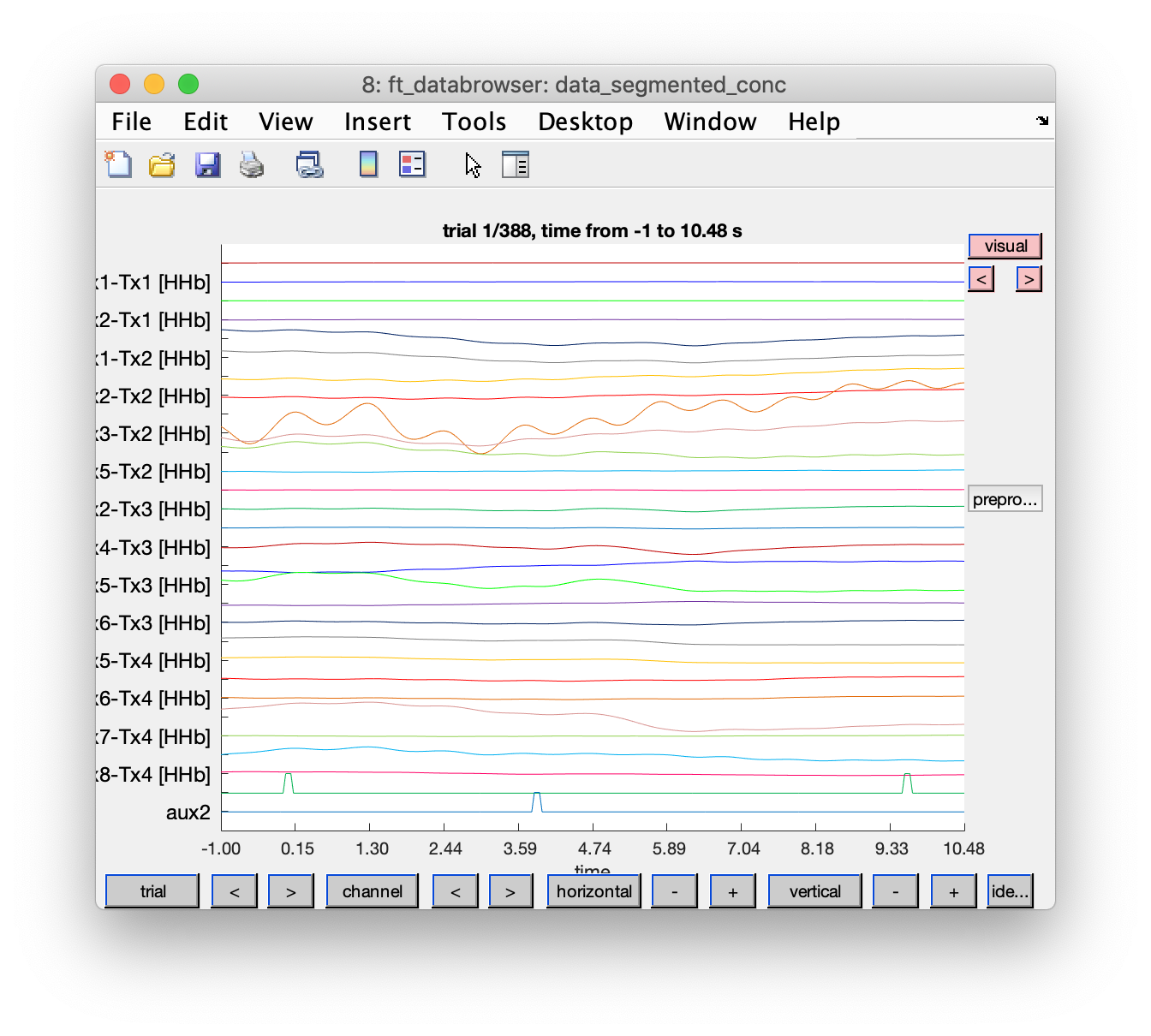We can make selective averages by specifying `cfg.trials`

``````cfg = [];
cfg.trials = ismember(data_segmented_conc.trialinfo, [11 12 13 14 15]); % average over all presented sentences
timelock1 = ft_timelockanalysis(cfg, data_segmented_conc);
cfg.trials = ismember(data_segmented_conc.trialinfo, [21 22 23 24 25]); % average over all responses
timelock2 = ft_timelockanalysis(cfg, data_segmented_conc);

cfg = [];
cfg.layout = 'ordered';
cfg.columns = 6; % use an even number to keep the HbO and hBR together
cfg.skipcomnt = 'no';
cfg.skipscale = 'no';
cfg.mychan = {'aux1', 'aux2'};
cfg.mychanscale = [100000 100000];
cfg.interactive = 'no';
cfg.showlabels = 'yes';
ft_multiplotER(cfg, timelock1, timelock2)
``````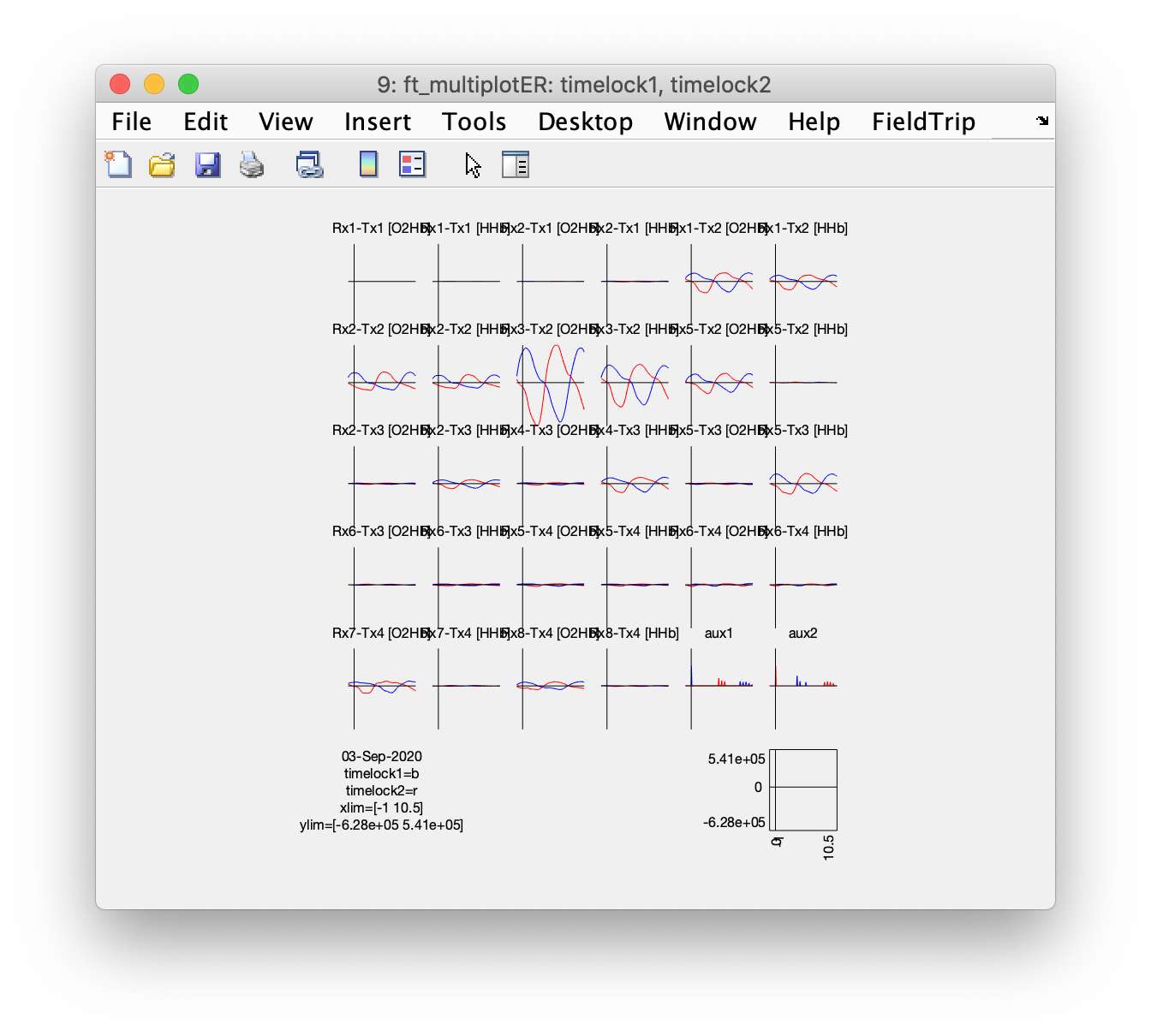In the lower right we can see the average of the “aux1” and “aux2” channels, which contain the TTL pulses. It is clear that due to the long segment that we selected for each trial, not only the stimulus related activity is included, but also the reposnee related activity (which is jittered a bit). We can even see the onset of the stimulus in the next next trial at the end of each segment.

There are a number of channels that show a clear up-down deflection. Since the experimental design consists of a continuous sequence of stimulus-response-stimulus-response-etc, it is hard to tell whether we have stimulus related activity or response related activity, or both. Even worse for the interpretation is that the optodes are placed over the temporal region of the head and that the subjects were responding with overt speack, which meant that they made regular jaw and head movements. Without further information about the experiment it is hard to rule out that the observed sequence of responses is not merely due to a repetetive movement or muscle artifact.

Although the intrepretation of the responses might be hard due to potentially confounding movement or muscle artifacts, we can continue and make condition specific averages.

``````cfg = [];
cfg.trials = data_segmented_conc.trialinfo==11;
timelock11 = ft_timelockanalysis(cfg, data_segmented_conc);
cfg.trials = data_segmented_conc.trialinfo==12;
timelock12 = ft_timelockanalysis(cfg, data_segmented_conc);
cfg.trials = data_segmented_conc.trialinfo==13;
timelock13 = ft_timelockanalysis(cfg, data_segmented_conc);
cfg.trials = data_segmented_conc.trialinfo==14;
timelock14 = ft_timelockanalysis(cfg, data_segmented_conc);
cfg.trials = data_segmented_conc.trialinfo==15;
timelock15 = ft_timelockanalysis(cfg, data_segmented_conc);

cfg = [];
cfg.layout = 'ordered';
cfg.columns = 6; % use an even number to keep the HbO and hBR together
cfg.skipcomnt = 'no'; % this is for ft_prepare_layout
cfg.skipscale = 'no'; % this is for ft_prepare_layout
cfg.showcomnt = 'yes';
cfg.showscale = 'yes';
cfg.mychan = {'aux1', 'aux2'};
cfg.mychanscale = [100000 100000];
cfg.interactive = 'yes';
cfg.showlabels = 'yes';
cfg.ylim = 'maxabs';
cfg.baseline = [-0.1 -0.1]; % just before the TTL pulse in the aux channels
ft_multiplotER(cfg, timelock11, timelock12, timelock13, timelock14, timelock15)
``````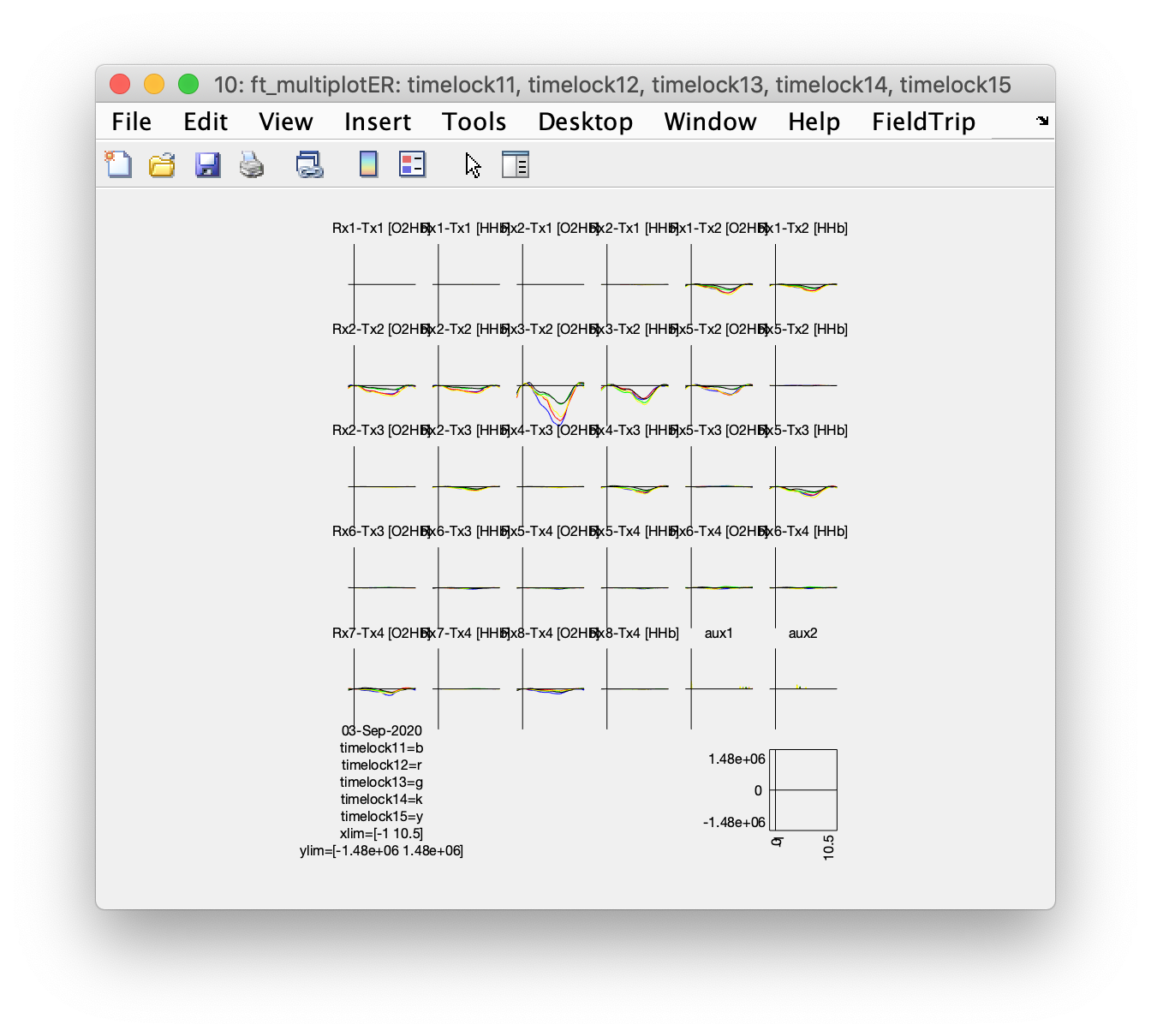After baseline correcting (which is done while making the figure, it could also have been done using ft_preprocessing) we can see condition differences in the event-related responses in the 5 conditions. However, due to the alternating stimulus-response sequence and the response possibly being confounded with an artifact, it is hard to tell whether the difference is due to the stimulus or to the response that could affect the baseline of each subsequent trial.

## Closing remarks

Looking at some more datasets, I noticed that the dataset `S1001_run01.nirs` contains overall clean data. The data for subject 2 (`S1002_run01.nirs`) is not shared. The data of subject 3 `S1003_run01.nirs` has some movement(?) artifacts, but also a channel that seems to show a response on every “aux1” stimulus. It would be interesting to follow up these analyses with a more detailled look into the time-locked and the spontaneous artifacts that can be observed in the data.